# r.d/b.x

1.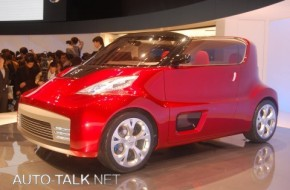### Nissan R.D/B.X

This compact sedan features a direct-injection gasoline engine with a continuously variable transmission for maximum efficiency in a futuristic four-seater.
2.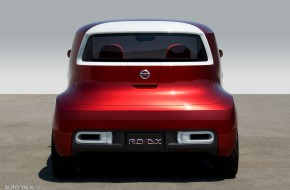### Nissan Round Box (R.D/B.X)

Nissan Round Box (R.D/B.X)
3.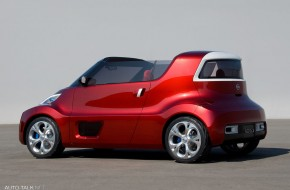### Nissan Round Box (R.D/B.X)

Nissan Round Box (R.D/B.X)
4.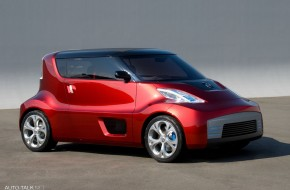### Nissan Round Box (R.D/B.X)

Nissan Round Box (R.D/B.X)
5.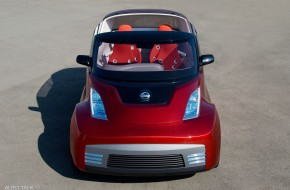### Nissan Round Box (R.D/B.X)

Nissan Round Box (R.D/B.X)
6.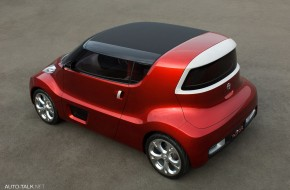### Nissan Round Box (R.D/B.X)

Nissan Round Box (R.D/B.X)
7.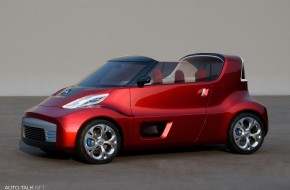### Nissan Round Box (R.D/B.X)

Nissan Round Box (R.D/B.X)
8.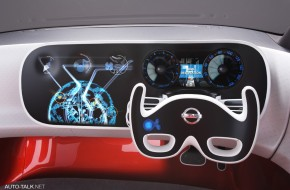### Nissan Round Box (R.D/B.X)

Nissan Round Box (R.D/B.X)
9.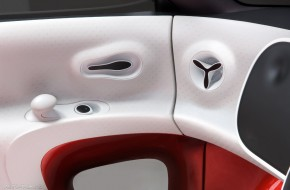### Nissan Round Box (R.D/B.X)

Nissan Round Box (R.D/B.X)
10.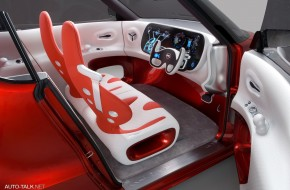### Nissan Round Box (R.D/B.X)

Nissan Round Box (R.D/B.X)
11.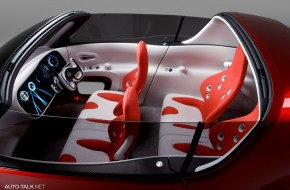### Nissan Round Box (R.D/B.X)

Nissan Round Box (R.D/B.X)
12.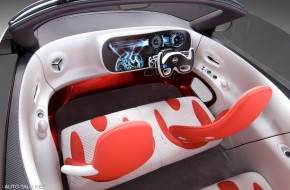### Nissan Round Box (R.D/B.X)

Nissan Round Box (R.D/B.X)
13.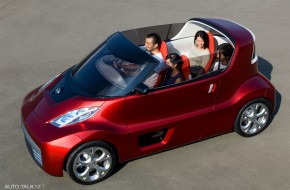### Nissan Round Box (R.D/B.X)

Nissan Round Box (R.D/B.X)
14.### Nissan Round Box (R.D/B.X)

Nissan Round Box (R.D/B.X)
15.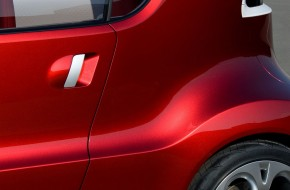### Nissan Round Box (R.D/B.X)

Nissan Round Box (R.D/B.X)
16.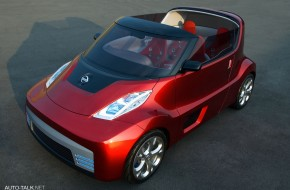### Nissan Round Box (R.D/B.X)

Nissan Round Box (R.D/B.X)
17.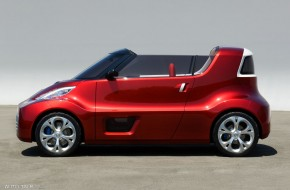### Nissan Round Box (R.D/B.X)

Nissan Round Box (R.D/B.X)
18.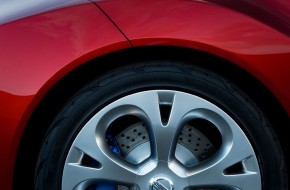### Nissan Round Box (R.D/B.X)

Nissan Round Box (R.D/B.X)
19.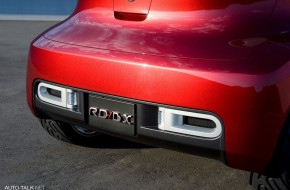### Nissan Round Box (R.D/B.X)

Nissan Round Box (R.D/B.X)
20.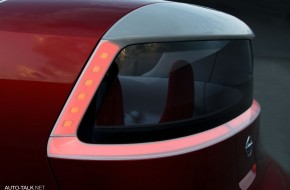### Nissan Round Box (R.D/B.X)

Nissan Round Box (R.D/B.X)